International
Tables for
Crystallography
Volume B
Reciprocal space
Edited by U. Shmueli

International Tables for Crystallography (2010). Vol. B, ch. 1.3, p. 44   | 1 | 2 |

## Section 1.3.2.6.7. The Poisson summation formula

G. Bricognea

aGlobal Phasing Ltd, Sheraton House, Suites 14–16, Castle Park, Cambridge CB3 0AX, England, and LURE, Bâtiment 209D, Université Paris-Sud, 91405 Orsay, France

#### 1.3.2.6.7. The Poisson summation formula

| top | pdf |

Let, so that. Let R be the lattice distribution associated to lattice Λ, with period matrix A, and letbe associated to the reciprocal lattice. Then we may write: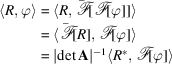i.e.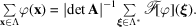This identity, which also holds for, is called the Poisson summation formula. Its usefulness follows from the fact that the speed of decrease at infinity of ϕ andare inversely related (Section 1.3.2.4.4.3), so that if one of the series (say, the left-hand side) is slowly convergent, the other (say, the right-hand side) will be rapidly convergent. This procedure has been used by Ewald (1921)[see also Bertaut (1952), Born & Huang (1954)] to evaluate lattice sums (Madelung constants) involved in the calculation of the internal electrostatic energy of crystals (see Chapter 3.4in this volume on convergence acceleration techniques for crystallographic lattice sums and Chapter 3.5on modern extensions of the Ewald summation method).

When ϕ is a multivariate Gaussianthen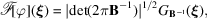and Poisson's summation formula for a lattice with period matrix A reads: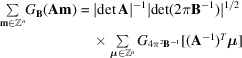or equivalently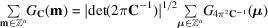with### References

Bertaut, E. F. (1952). L'énergie électrostatique de réseaux ioniques. J. Phys. Radium, 13, 499–505.
Born, M. & Huang, K. (1954). Dynamical Theory of Crystal Lattices. Oxford University Press.
Ewald, P. P. (1921). Die Berechnung optischer und electrostatischer Gitterpotentiale. Ann. Phys. Leipzig, 64, 253–287.